### Interesting elasticity, especially spring elasticity

Here we have four examples of elastic behaviour in mechanics. We suppose absolutely rigid the ball, the wall and the construction. Reference system is outside – connected with “paper”. See images below.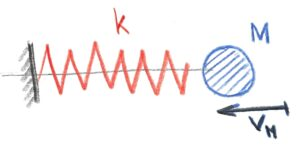The first example is clear – a ball with mass M1 moving with velocity vM1 against a fixed rigid wall with a spring of stiffness k.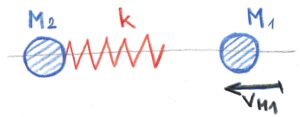The second example is similar to the first with the important difference that the fixed stationary wall has disappeared and instead there is a standing ball with mass M2.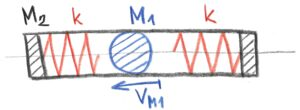In the third and fourth examples, a ball with mass M1 is enclosed in a rigid closed construction with mass M2
The third and fourth examples look the same, but are not the same. The difference between initial conditions.
In the third example, the ball with mass M1 has an initial velocity vM1, while the closed rigid construction with mass M2 is standing. Both left and right springs with the same stiffness k are free – not compressed.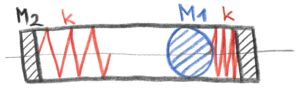In the fourth case, both the ball with mass M1 and the structure with mass M2 are initially standing. The right spring with stiffness k is maximally compressed. The left spring with stiffness k is free.

Next, we will solve the measurement of motion in the previously mentioned examples with respect to the reference system. This is in the case where we replace the photons with mechanical balls of mass m with velocity v. The problem is the so-called rest mass of the bodies. We know very well that there is no ideal rest system in the world. Neither is an ideal rest mass.In the first case we have to solve the problem of ball impact to spring. To solve the duration of impact, the maximall compress of spring and, if we please, the progression of velocity and acceleration of the ball. Firstly I must write – to solve upper case is impossible in the real world. We must this case make easy – to simplify it. To take out the case from the world. To idealize the case. Ignore all irrelevant details. But the case is given by the world. This case can not exist without the world in the same way like organisms – tear off the flower and study it, especially its living processes. Nothing against simplification, but don´t remember it our model has its own limitations.

Initial conditions:
The ball with mass M with inital velocity v in the direction of the axis x of the spring, the weightless spring with a stiffness k, the fixed wall – it is also our reference point.

To solve only max. compress of the spring – it´s easy.
We use only two fromulas – first of them for kinetic energy Ek of the moving ball Ek=1/2Mv^2

the second one for potential energy Ep of compressed spring
Ep=1/2kx^2

from the law of conservation energy Ek = Ep after that we are able to solve the max. compress x max. of the spring.

the solve the duration of impact and the progress of velocity or deccelleration and accelleration we must use the classical mechanics consideration about the force between ball and the spring

the inertia force Fi = M*a

the “spring” force Fs = – k*x

these two forces are equall through the duration of the impact

Fi = Fs  then M*a = – k*x  or   M*a + k*x = 0

we are able to solve the accelleration a of the ball at any distance x from the beginning of the impact – we must use numerical methods, in the other hand to solve uppper case analytically we must use differential equations – where m represents mass M of the ball, a – accelleration, x – distance, k – stiffness, t – time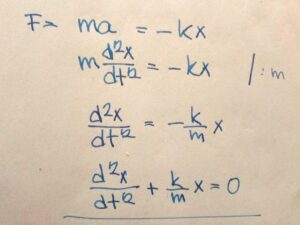The results: functional dependence of distance on time x = f(t), x = C cos Ω t, where Ω^2= k/m and Ω*t=2*π . From here we can calculate the duration t of the impact.
The angular velocity can also be expressed in units of seconds. Instead of the unit radian for angle, we use 1 as the unit of angle. In that case 1 means 360 degrees or 2*π radians. Thus angle 90° (π/2) will be 1/4,  then  angle 180° (π)  will be 1/2 and  angle 270° (3/4*π)  will be only 3/4 and finally the angle 360° (2*π) will be 1. So here we are at rounds per second. Generally cycles per seconds. A second, however, is defined as a certain number of wavelengths of selected radiation that serves as the basic unit of time. Then cycles of anything per base unit of time. For example, the number of different wavelengths per 1 selected (metric) wavelength. Or the number of events per 1 wavelength.

In the end we have got the succesfull model with results and, no doubt, such results are verified by measurement. What to wish for more. To solve the problem of elasticity? To find out hidden processes with verified model. To use our model for recognition of hidden processes connected with elasticity? Elasticity is the base of the universe. Without elasticity there could be only singularities (to close 0 or ∞ ). But elasticity needs a space. And the space needs matter – without matter there is no empty space. And matter (excitations of quantum field) needs time. And matter has the weight. To explain the subject of spacetimematter with our simplified models? Nothing against idealization, otherwise we wouldn’t do anything. We have to start somehow. But we need to go further. Correction about the unknown. That is the meaning of knowledge.

to be continued next time …

Technical drawings with a lot of mechanical devices. Steam engines, turbines, motors, etc.